Click to Chat

1800-1023-196

+91-120-4616500

CART 0

• 0

MY CART (5)

Use Coupon: CART20 and get 20% off on all online Study Material

ITEM
DETAILS
MRP
DISCOUNT
FINAL PRICE
Total Price: Rs.

There are no items in this cart.
Continue Shopping```System of Linear Equations

System of Linear EquationsWe have already discussed the linear equations under the topic Quadratic Equations. The set of n (> 2) linear equations is called the system of linear equations and this system is said to be consistent if it has at least one solution.

Illustration:

Check the consistency of the following system of equation.

(i)     x + 2x = 4

2x + 4y = 9

This is inconsistent because it has no solution i.e. there is no value of x and y which satisfies both the equations.

(ii)    x + y = 4

X - y = 0

This is consistent because it has a solution x = 2 and y = 2

Solving System of Linear Equations by using Determinants

There are several methods to solve the system of linear equations but determinant is one of the best mathematical tool from which we can solve the system of linear equations very easily.

CRAMER'S RULE

Case I:      System of linear equations in two variables.

Let, us have the system of equations

a1x + b1 y + c1 = 0

a2x + b2 y + c2 = 0, where a1/a2 ≠ b1/b2

Solving by cross multiplication, we get,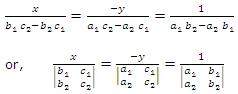Case II:    System of linear equations in three variables:

Let, us have the system of equations.

a1 x + b1 y + c1 = d1

a2 x + b2 y + c2 = d2

a3 x + b3 y + c3 = d3.·.    x = Δ1/Δ

Similarly

y = Δ2/Δ and z = Δ3/Δ, where Δ ≠ 0.

Illustration:

Solve the following system of equations.

2x - y + 3z = 9

x + y + z = 6

x - y + z = 2

Solution:

Here Δ =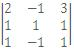= -2 (≠0)

Δ1 == -2

Δ2 =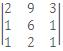= -4

Δ3 == -6

By Cramer's Rule,

x = Δ1/Δ = 1

y = Δ2/Δ = 2

z = Δ3/Δ = 3

Consistency of the System of the Equations

Let the given system of equation be

a1 x + b1 y + c1 = d1

a2 x + b2 y + c2 = d2

a3 x + b3 y + c3 = d3

From the last enquiry,Now, there are two possibilities.

Case I.      when Δ ≠ 0

In this case we have,

x = Δ1/Δ, y = Δ2/Δ, z = Δ3/Δ = 3

Hence unique value of x, y, z will be obtained

Case II:   when Δ = 0

(a)  When at least one of Δ1, Δ2 and Δ3 is non zero.

Let Δ1 ¹ 0, then from case I, Δ1 = x Δ  will not be satisfied for any value of x because Δ = 0 and Δ1 ¹ 0 and hence no value of x is possible in this case. Similarly when Δ2 ¹ 0, and Δ2 = y Δ and similarly for Δ3 ¹ 0.

Therefore, if Δ = 0 and any of Δ1, Δ2 and Δ3 is non zero, then no solution is possible and hence system of equations will be inconsistent.

(b)  When Δ = 0 and Δ1 = Δ2 = Δ3 = 0.

and we have,

Δ1 = x Δ, Δ2 = y Δ and Δ3 = zΔ will be true for all values of x, y and z. But since a x + b1 y + c1 z = d1, so, only two of x, y, z will be independent and third will be dependent on other two, therefore if Δ = Δ1 = Δ2 = Δ3 = 0, then the system of equations will be consistent and it will have infinitely many solutions.

System of homogeneous linear equations

A system of linear equations is said to be homogenous if sum of the powers of the variables in each term is same. In other words we can say that if constant term is a zero in a system of linear equations.

Let's consider the system of linear homogeneous equations to be

a1 x + b1 y + c1 z = 0

a2 x + b2 y + c2 z = 0

a3 x + b3 y + c3 z = 0

By clean observation, x = 0, y = 0, z = 0 is a solution of above system of equations. This solution is known as trivial solution. For non-trivial solution, consider first two equations from above system.

x/(b1 c2-b2 c1 ) = y/(c1 a2-c2 a2 ) = z/(a1 b2-b1 a2 ) = k

.·.     x = k (b1 c2 - b2 c1), y = k (c1 a2 - c2 a1), z = k (a1 b2 - b2 a2)

Now putting these values in the third equation, we get,

k[a3 (b1 c2 - c1 b2) -b3 (a1 c2 - c1 a2) -c3 (a1 b2 - b1 a2)] = 0

or, a3 (b1 c2 - c1 b2) -b3 (a1 c2 - c1 a2) -c3 (a1 b2 - b1 a2) = 0 [k ≠ 0]

or,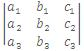= 0

This is required condition for the above system of above homogeneous linear equations to have non-trivial solution.

Illustration:

For what value of k doe the following homogeneous system of equations posses a non-trivial solution: x + ky + 3z = 0, kx + ky - 2z = 0, 2x + 3y - 4z = 0.

Solution:

For non-trivial solution i.e. D = 0.

D == 0.

Apply R2 - 3R1 and R3 - 2R1 =>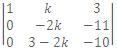= 0.

Expanding by 1st column, we get k = 33/2.

Illustration:

For what values of p and q, the system of equation 2x + py + 6z = 8, x + 2y + qz = 5, x + y + 3z = 4 has (i) no solution (ii) a unique solution (iii) infinitely many solutions.

Solution:

Here Δ == 2(6-q) - p(3-q) + 6(1-2) = 12 - 2q - 3p + pq - 6

=pq-2q-3p+6 = (p-2)(q-3). Also Δ1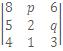6(6-p)-p(15-49)+6(5-8)

= 48 - 8q - 15p + 4pq - 18 = 4pq - 18 = 4pq - 8q - 15p + 30 = 4q(p-2)
- 15(p - 2) = (4q - 15)(p - 2), Δ2 =2(15-4q)-8(3-q)+6(4-5)=0.

and Δ3 =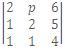= 2(8 - 5) - p(4 - 5) + 8(1 - 2) = p - 2.

Case-1: When Δ ≠ 0, i.e. p ≠ 2, q ≠ 3, given system of equation has a unique solution.

Case-II: When Δ = 0, i.e. p = 2, or q = 3.

When p = 2, Δ = 0, Δ1 = 0, Δ2 = 0, Δ3 = 0.

=> given system of equation has infinitely many solutions.

When q = 3, p ≠ 2, D = 0, D1 ≠ 0. The system has no solution.

You can join live online classroom programmes for one year and two year and be a winner in the upcoming IIT JEE, AIEEE and other engineering examination. We also provide Study material and you can come and read them free of cost from home just by visiting askIITians.com.

To read more, Buy study materials of Matrices and Determinants comprising study notes, revision notes, video lectures, previous year solved questions etc. Also browse for more study materials on Mathematics here.
```### Course Features

• 731 Video Lectures
• Revision Notes
• Previous Year Papers
• Mind Map
• Study Planner
• NCERT Solutions
• Discussion Forum
• Test paper with Video Solution